# scipy.spatial.distance.sokalmichener¶

scipy.spatial.distance.sokalmichener(u, v)[source]

Computes the Sokal-Michener dissimilarity between two boolean 1-D arrays.

The Sokal-Michener dissimilarity between boolean 1-D arrays u and v, is defined as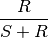where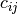is the number of occurrences of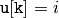and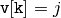for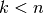,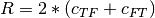and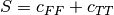.

Parameters : u : (N,) array_like, bool Input array. v : (N,) array_like, bool Input array. sokalmichener : double The Sokal-Michener dissimilarity between vectors u and v.

#### Previous topic

scipy.spatial.distance.seuclidean

#### Next topic

scipy.spatial.distance.sokalsneath